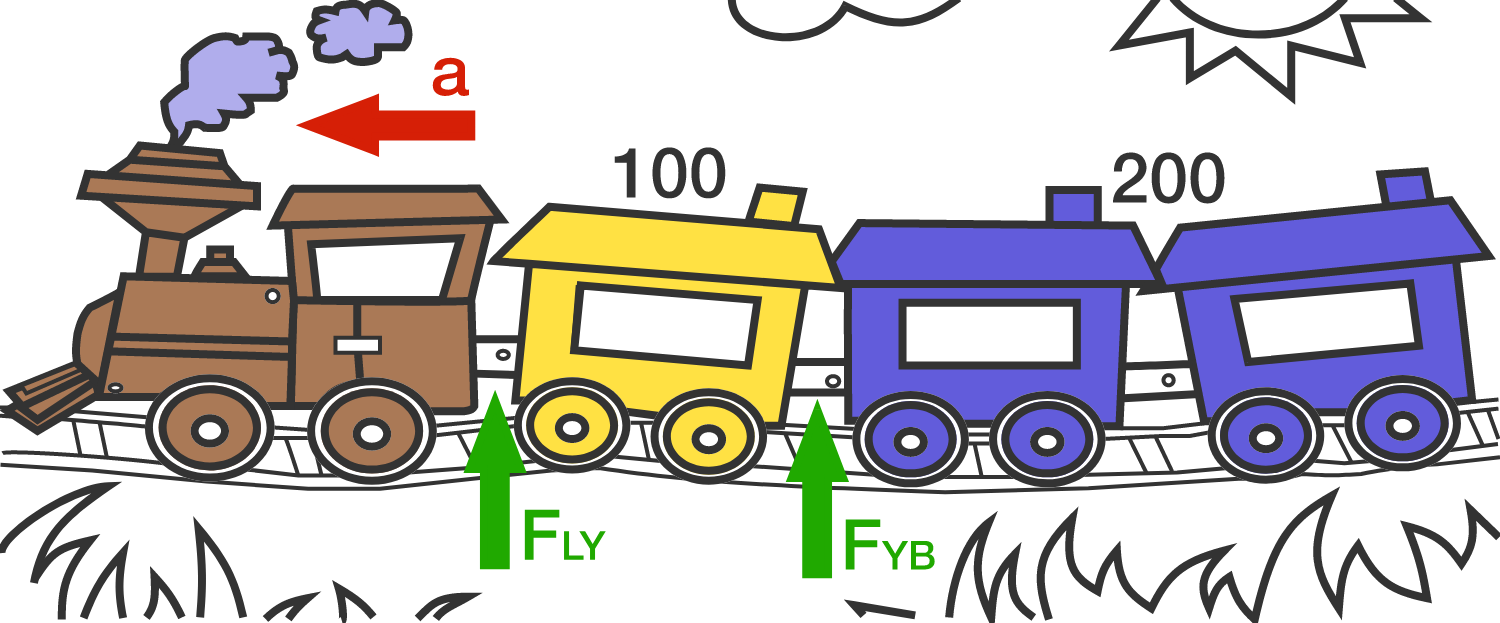# Two-color Train

A train consists of a locomotive, pulling 100 yellow cars, which in turn pull 200 blue cars. The train travels on a level track, accelerating forward at rate $a$.Each yellow car has 3 times as much mass as a blue car.

The cars experience rolling friction opposing the motion: $F_f = \mu m g$, where $m$ is the mass of the car, $\mu = 0.10$ for yellow cars, and $\mu = 0.20$ for blue cars.

Let $F_{LY}$ be the force exerted by the locomotive on the first yellow car, and $F_{YB}$ the force by the last yellow car on the first blue car.

If $F_{YB} = \tfrac12\ F_{LY}$, how fast does the train accelerate? Give your answer as the ratio $\frac{a}{g}$.

×